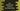# How to find the sum of two numbers by using a class in C++## How to find the sum of two numbers by using a class in C++:

Classes can help us in many ways. We can create a class and objects of that class can be used in different ways. In this example, I will show you how to create a Class to find the sum of different numbers in C++.

We will create a class that can hold two numbers and it will have different methods to find the sum and print the value.

Once the class is created, we will create different objects for that class and find the sum for different numbers.

With this program, you will learn how to create Classes, how to use constructors in a class, how to use methods in a class etc.

Let’s have a look at the program:

## C++ program to find the sum of two numbers by using a class:

Below program uses a class to find the sum of two numbers:

``````#include <iostream>
#include <string>

using namespace std;

class Calculator
{
private:
int a, b, sum;

public:
Calculator(int first, int second)
{
a = first;
b = second;
}

void findSum()
{
sum = a + b;
}

void printSum()
{
cout << a << " + " << b << " = " << sum << endl;
}
};

int main()
{
Calculator c1(1, 2), c2(11, 23), c3(33, 123);

c1.findSum();
c2.findSum();
c3.findSum();

c1.printSum();
c2.printSum();
c3.printSum();

return 0;
}``````

Here,

• Calculator is the class we are using in this program to find the sum of two numbers.
• It has three private integer variables: a, b, and sum. We can’t access these variables from an object because these are private variables.
• It also has two public methods and one constructor.

• findSum is a public method. It doesn’t take any parameter and it returns nothing. It only finds the sum of the numbers and stores it in the variable sum.
• printSum is another public method. It also doesn’t take any parameter and it returns nothing. This method prints the value of a, b and sum of the numbers i.e. the value of sum variable.
• In main, we are creating three Calculator objects c1, c2 and c3. Each one is created by passing different numbers to the constructors.
• We are calling the findSum method on these objects. This will calculate the sum of the variables and store it inside these objects.
• Then, we are calling the printSum method on these objects again to print the sum values.

If you run the above program, it will print the below output:

``````1 + 2 = 3
11 + 23 = 34
33 + 123 = 156``````

As you can see here, all of these lines are printed for different objects we have created.

One thing you should note that we have two methods here: findSum and printSum. findSum is used to find the sum of the numbers and printSum is used to print the sum. We should always call printSum before findSum. This is because printSum is used to print the sum value and findSum is used to find the sum value. We can’t print the sum before it is calculated.

So, always make sure to call findSum for an object before you call printSum.